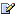CTK Exchange Front Page Movie shortcuts Personal info Awards Reciprocal links Terms of use Privacy Policy Cut The Knot! MSET99 Talk Games & Puzzles Arithmetic/Algebra Geometry Probability Eye Opener Analog Gadgets Inventor's Paradox Did you know?... Proofs Math as Language Things Impossible My Logo Math Poll Other Math sit's Guest book News sit's Recommend this siteCTK Exchange

 Subject: "Mahavira's formula for cyclic quadrilaterals" Previous Topic | Next Topic
 ConferencesThe CTK ExchangeCollege mathTopic #612Printer-friendly copyEmail this topic to a friend Reading Topic #612
meleftheguest
Apr-11-07, 07:21 PM (EST)

 Does anyone know the proof of Mahavira's formulae for the calculation of the inner diagonals of the cyclic quadrilaterals? Presumably, they must be a continuation of Ptolemy's theorem. They are:x=Sq.Root<(ad+bc)/(ab+cd)x(ac+bd)> and symmetrically for y.Thanks.

Subject     Author     Message Date     IDMahavira's formula for cyclic quadrilaterals meleftheApr-11-07 TOPRE: Mahavira's formula for cyclic quadrilaterals Pierre Charland May-11-07 1RE: Mahavira's formula for cyclic quadrilaterals meleftheMay-19-07 2RE: Mahavira's formula for cyclic quadrilaterals alexb May-19-07 3RE: Mahavira's formula for cyclic quadrilaterals meleftheMay-20-07 4RE: Mahavira's formula for cyclic quadrilaterals alexb May-20-07 5RE: Mahavira's formula for cyclic quadrilaterals Pierre Charland May-27-07 6

 Conferences | Forums | Topics | Previous Topic | Next Topic
Pierre Charland
Member since Dec-22-05
May-11-07, 06:40 AM (EST)1. "RE: Mahavira's formula for cyclic quadrilaterals"
In response to message #0

 I found a partial proof inHoward Eves, Great Moments in Mathematics Before 1650, 10.5 p.108Let ABCD be a cyclic quadrilateral of diameter t.Let BD=m and AC=n.Let theta = the angle between either diagonal and the perpendicular upon the other.Then, (using triangle's formula ab=2hR applied to DAB and DCB), we getmt cos theta = ab+cdnt cos theta = ad+bcSo m/n = (ab+cd)/(ad+bc)Also mn = ac+bd (Ptolemy relation)Multiplying those last 2 equations, we get:m^2=(ab+cd)(ac+bd)/(ad+bc)Dividing instead, we get:n^2=(ac+bd)(ad+bc)/(ab+cd)===========================I also found some info there:https://www.pballew.net/cycquad.htmlhttps://www.vias.org/comp_geometry/geom_quad_cyclic.htmlhttps://www.answers.com/topic/mahavira-mathematicianAlphaChapMtlmeleftheguest
May-19-07, 03:49 PM (EST)

2. "RE: Mahavira's formula for cyclic quadrilaterals"
In response to message #1

 I do not understand it unfortunately. What formula are you referring to? What quantity is m.t.cosČ ? Could you please explain?alexb
Charter Member
2013 posts
May-19-07, 03:56 PM (EST)3. "RE: Mahavira's formula for cyclic quadrilaterals"
In response to message #2

 Have a look athttps://www.cut-the-knot.org/proofs/ptolemy.shtml#MahaviraIt's the same as Pierre's remark but perhas a little more detailed. What I want you to do is to make a diagram where you mark the angle theta and think of what cos(theta) signifies, OK?meleftheguest
May-20-07, 10:28 AM (EST)

4. "RE: Mahavira's formula for cyclic quadrilaterals"
In response to message #3

 Dear Alex,Sorry, still nothing! I do not understand anything! Are you sure there is not a flaw in the argument? I have a feeling there is. Could you please send me a diagram and an explanation? You may draw it by hand and fax it through at +30-210-7219225 if this is easier for you.The formula is proven very easily by using the cosine rule.m^2=b^2+c^2-2.b.c.cosC =a^2+d^2-2.a.d.cosA=a^2+d^2-2.a.d.cos(180-C)=a^2+d^2+2.a.d.cosCMultiply the first with ad and the second with bc, add them up and you will arrive at m^2=(ab+cd).(ac+bd)/(ad+bc)I am really surprised. I do not understand what cosč signifies. There might be an error in the proof.Have a bash at the probability question I posed. I think I have got an answer, it depends on what one is really asking. I look forward to see the replies.alexb
Charter Member
2013 posts
May-20-07, 04:59 PM (EST)5. "RE: Mahavira's formula for cyclic quadrilaterals"
In response to message #4

 ab = hR - can you verify that? It'says that in a triangle the product of two sides equals the product of the diameter circumscribed circle and the altitude to the third side.In a quadrilateral, you have two pairs of triangles sharing a base (the third side in 1). Bases are the diagonals of the quadrilateral. Consider one pair at a time, say, ab = ht and cd = gt, where h is the altitude to from the ab vertex to the diagonal m, I belive. g is the altitude from the cd vertex to the sam diagonal. Each of these triangles contains a piece of the diagonal n which plays the hypotenuse, one in a triangle with side h, the other in a triangle with side g. The triangles are similar. You can writeh = cos(theta)×(one piece) andg = cos(theta)×(the other piece).On summing up you geth + g = cos(theta)·nThus adding ab = ht and cd = gt gives yount·cos(theta) = ab + cd.Sorry if I confused m and n.Pierre Charland
Member since Dec-22-05
May-27-07, 06:43 PM (EST)6. "RE: Mahavira's formula for cyclic quadrilaterals"
In response to message #2

 sorry for my late reply,the angle theta is the angle between either diagonal and the perpendicular upon the other.Four of them are marked is the included image.AlphaChapMtl

 Conferences | Forums | Topics | Previous Topic | Next Topic
 Select another forum or conference Lobby The CTK Exchange (Conference)   |--Early math (Public)   |--Middle school (Public)   |--High school (Public)   |--College math (Public)   |--This and that (Public)   |--Guest book (Protected)   |--Thoughts and Suggestions (Public) Educational Press (Conference)   |--No Child Left Behind (Public)   |--Math Wars (Public)   |--Mathematics and general education (Public)You may be curious to have a look at the old CTK Exchange archive.